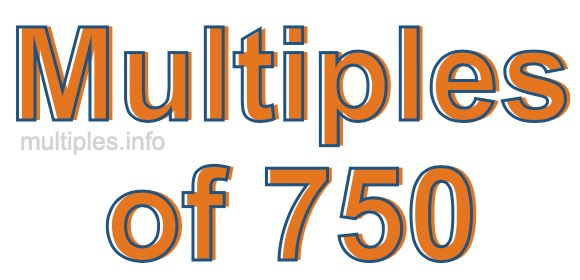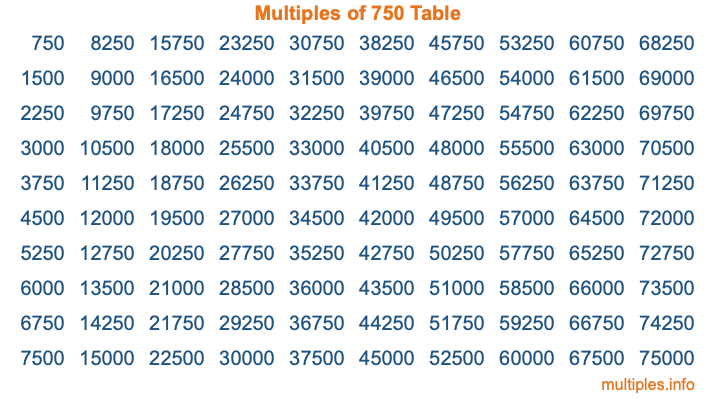Multiples of 750Welcome to the Multiples of 750 page. Here we will first teach you everything you will ever need to know about the multiples of 750, and then give you a study guide summary of everything we taught you to make sure you remember it all. Use this page to look up facts and learn information about the multiples of 750. This page will make you a multiples of seven hundred fifty expert!

Definition of Multiples of 750
Multiples of 750 are all the numbers that when divided by 750 equal an integer. Each of the multiples of 750 are called a multiple. A multiple of 750 is created by multiplying 750 by an integer.

Therefore, to create a list of multiples of 750, you start with 1 multiplied by 750, then 2 multiplied by 750, then 3 multiplied by 750, and so on for as long as you want. Thus, the list of the first five multiples of 750 is 750, 1500, 2250, 3000, and 3750. To see a larger list of multiples of 750, see the printable image of Multiples of 750 further down on this page. We also have a category where you can choose any nth multiple of 750.

Multiples of 750 Checker
The Multiples of 750 Checker below checks to see if any number of your choice is a multiple of 750. In other words, it checks to see if there is any number (integer) that when multiplied by 750 will equal your number. To do that, we divide your number by 750. If the the quotient is an integer, then your number is a multiple of 750.

Is  a multiple of 750?

Least Common Multiple of 750 and ...
A Least Common Multiple (LCM) is the lowest multiple that two or more numbers have in common. This is also called the smallest common multiple or lowest common multiple and is useful to know when you are adding our subtracting fractions. Enter one or more numbers below (750 is already entered) to find the LCM.

Check out our LCM Calculator if you need more details about the Least Common Multiple or if you need the LCM for different numbers for adding and subtraction fractions.

nth Multiple of 750
As we stated above, 750 is the first multiple of 750, 1500 is the second multiple of 750, 2250 is the third multiple of 750, and so on. Enter a number below to find the nth multiple of 750.

th multiple of 750

Multiples of 750 vs Factors of 750
750 is a multiple of 750 and a factor of 750, but that is where the similarities end. All postive multiples of 750 are 750 or greater than 750. All positive factors of 750 are 750 or less than 750.

Below is the beginning list of multiples of 750 and the factors of 750 so you can compare:

Multiples of 750: 750, 1500, 2250, 3000, 3750, etc.

Factors of 750: 1, 2, 3, 5, 6, 10, 15, 25, 30, 50, 75, 125, 150, 250, 375, 750

As you can see, the multiples of 750 are all the numbers that you can divide by 750 to get a whole number. The factors of 750, on the other hand, are all the whole numbers that you can multiply by another whole number to get 750.

It's also interesting to note that if a number (x) is a factor of 750, then 750 will also be a multiple of that number (x).

Multiples of 750 vs Divisors of 750
The divisors of 750 are all the integers that 750 can be divided by evenly. Below is a list of the divisors of 750.

Divisors of 750: 1, 2, 3, 5, 6, 10, 15, 25, 30, 50, 75, 125, 150, 250, 375, 750

The interesting thing to note here is that if you take any multiple of 750 and divide it by a divisor of 750, you will see that the quotient is an integer.

Multiples of 750 Table
Below is an image of the first 100 multiples of 750 in a table. The table is in chronological order, column by column. The first column has the first ten multiples of 750, the second column has the next ten multiples of 750, and so on.The Multiples of 750 Table is also referred to as the 750 Times Table or Times Table of 750. You are welcome to print out our table for your studies.

Negative Multiples of 750
Although not often discussed or needed in math, it is worth mentioning that you can make a list of negative multiples of 750 by multiplying 750 by -1, then by -2, then by -3, and so on, to get the following list of negative multiples of 750:

-750, -1500, -2250, -3000, -3750, etc.

Multiples of 750 Summary
Below is a summary of important Multiples of 750 facts that we have discussed on this page. To retain the knowledge on this page, we recommend that you read through the summary and explain to yourself or a study partner why they hold true.

There are an infinite number of multiples of 750.

A multiple of 750 divided by 750 will equal a whole number.

750 divided by a factor of 750 equals a divisor of 750.

The nth multiple of 750 is n times 750.

The largest factor of 750 is equal to the first positive multiple of 750.

750 is a multiple of every factor of 750.

750 is a multiple of 750.

A multiple of 750 divided by a divisor of 750 equals an integer.

750 divided by a divisor of 750 equals a factor of 750.

Any integer times 750 will equal a multiple of 750.

Multiples of a Number
Here you can get the multiples of another number, all with the same attention to detail as we did for multiples of 750 on this page.

Multiples of
Multiples of 751
Did you find our page about multiples of seven hundred fifty educational? Do you want more knowledge? Check out the multiples of the next number on our list!